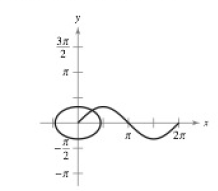Chapter 8.4, Problem 72E

Chapter
Section
Textbook Problem

# Arc length Show that the arc length of the graph of y = sin x on the interval [ 0 ,   2 π ] is equal to the circumference of the ellipse x 2 + 2 y 2 = 2 (see figure).To determine

To calculate: To show that the arc length of the graph of y=sin x on the interval x2+2y2=2 is equal to the circumference of the ellipse x2+2y2=2.

Explanation

Given: The graph of y=sin x on the interval x2+2y2=2 and the ellipse x2+2y2=2.

Refer to the question for graph.

Calculation:

For sin curve

(ds)2=(dx)2+(dy)20sds=02π1+(dydx)2dθ

Now for

sinθdydx=cosθs=102π1+cos2θdθ

Now for ellipse

Now for ellipse x2+2y2=2x22+y2=1a=2b=1x=sinθy=bsinθ(ds)2=(dx)2+(dy)2(ds)2=((acosθ)2+(bsinθ)2)

### Still sussing out bartleby?

Check out a sample textbook solution.

See a sample solution

#### The Solution to Your Study Problems

Bartleby provides explanations to thousands of textbook problems written by our experts, many with advanced degrees!

Get Started

#### In Exercises 49-62, find the indicated limit, if it exists. 56. limx2x+2x2

Applied Calculus for the Managerial, Life, and Social Sciences: A Brief Approach

#### 12. Is if?

Mathematical Applications for the Management, Life, and Social Sciences

#### Subtract: (10)(+4)

Elementary Technical Mathematics

#### True or False: is a convergent series.

Study Guide for Stewart's Multivariable Calculus, 8th